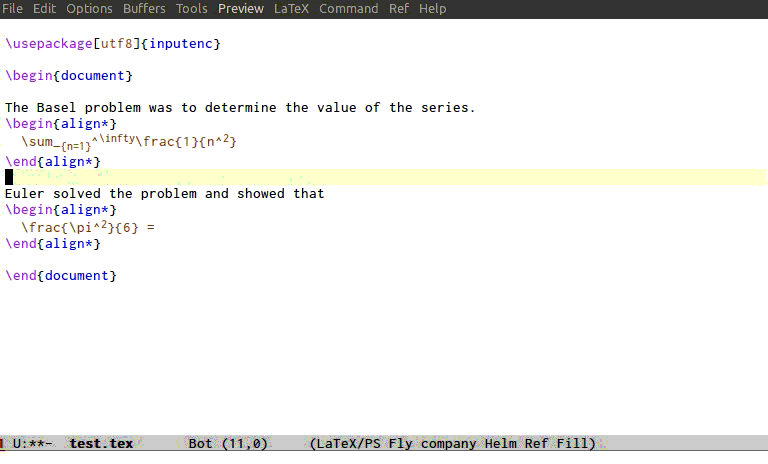It often happens that I need to copy some formula or equation and paste it as part of some other formula or equation (possibly with minor modifications). As a concrete example I have somewhere in my document $f(x)$ where f might be some complicated expression. Then somewhere else I have the partial expression $g()$ and now I would like to paste f(x) between the parenthesis to obtain $g(f(x))$.

This is of course easy to do by just selecting the part that I want. However the way moving around emacs buffers works, it is much easier1 to select and copy the whole expression $f(x)$ instead of just f(x). Then when pasting we will end up with $g($f(x)$)$, which is not what we want. If only there was a way to automatically remove the two $ from $f(x)\$ when pasting inside a math environment. Well, it is easy to modify the pasting command (called yank in Emacs) to do this automatically. Well, easy might be an overstatement. Let’s just say possible. Yes, possible is a better word.

If you do not want to read me ramble about how to do it and just want the modification you can get it here.

Let us get to it then. What do we need?

• First we need to figure out which Emacs command actually does the pasting. Well the user interface command for pasting is called yank. Just because calling it paste would be too ordinary and accessible. But yank itself just handles the interaction. The function that actually inserts is called insert-for-yank. It has a single argument, which is the string to be inserted. How does one find insert-for-yank I hear you ask? Obviously, one stares at the source code of the yank command.
So the idea is to advise the insert-for-yank function to precompose it with a function that will remove the desired math delimiters in the given string in cases when we are pasting inside a math environment.

• But, Aleš, I hear you ask, how do I know when I am pasting inside a math environment? Well to be precise, due to how broken amazing TeX is, detecting this in general statically is probably not possible due to pesky issues like undecidability.
But we do not care how to do it in general. LaTeX code is in general relatively structured and in any case, we are only interested in the common case, since the point is to make the common case more efficient, or at least less annoying.
Stop rambling then, and answer the question. Yes Master. Well, we will be sneaky. The good developers of AUCTeX have already thought about detecting when we are in math mode in connection with syntax highlighting, so we will just use that information. This does mean that font-lock-mode2 must be enabled (which is the default, I think). And who doesn’t use syntax highlighting anyhow?

Now let us put these two ingredients together.

First, the function which checks whether we are in math mode or not.

This function is pretty self-explanatory. We get the face of the character we are currently looking at and check whether it is the face that AUCTeX uses for displaying math. The second condition eliminates some corner cases, as explained in the comment. Checking whether we are looking at the right face is done using the auxiliary function latex/check-if-math-face.

The face of a character can be one of four things. We only check whether it is a symbol (in which case it must be the 'font-latex-math-face) or whether it is a list, in which case it is a list of faces, and one of those must be 'font-latex-math-face.

The function that does the main work is latex/remove-math-delims. It uses two auxiliary functions remove-newline-forward and remove-newline-backward to deal with redundant newlines that are typically left after removing \begin{...} and \end{...}. It also uses the function first-nsp-before and first-nsp-after which were introduced in the previous post.

The function is pretty self-explanatory, except for the two calls to latex/start-of-math-environment and latex/end-of-math-environment. These two check if we are currently looking at the beginning/end of a math environment. They are implemented as follows.

These two functions need the dash.el library function -keep. It is really just the filter function, but sadly standard Emacs does not come equipped with a good, functional, list library.

The final piece of the puzzle is the variable math-display-delims, which is exactly what is says. It is a list of math display environment deliminters. It should be a list of pairs, for example you could set it as

Finally, after all this suffering, we can advise the insert-for-yank function to use our specially crafted latex/remove-math-delims to strip math delimiters from the inserted string when it makes sense to do so. This is done as follows

That wasn’t difficult, was it? All praise Emacs the magnificent.

Here is a demo.1. Because one can use navigation commands like forward-sexp and forward-paragraph more easily.

2. It is probably™ called font-lock because something like syntax-highlighting-mode would be too easy to understand. One must be prepared to suffer to use Emacs.